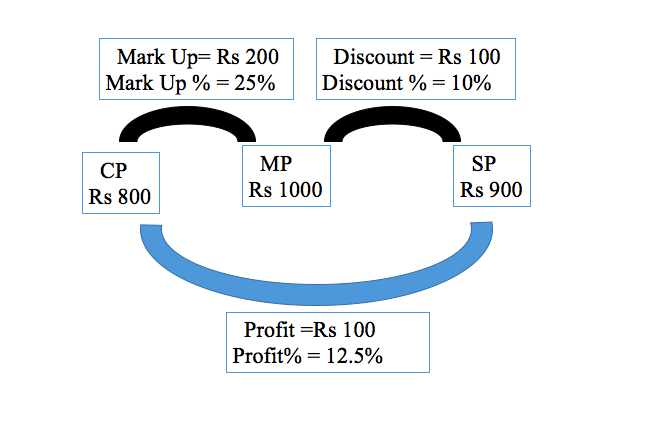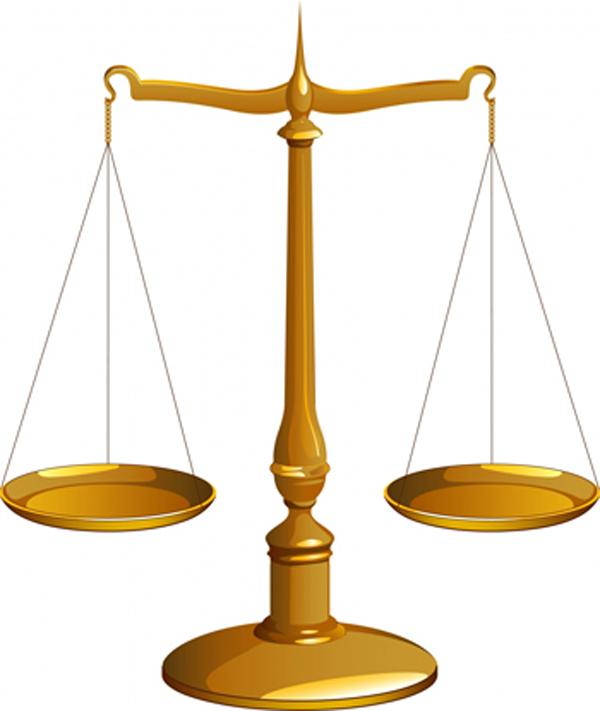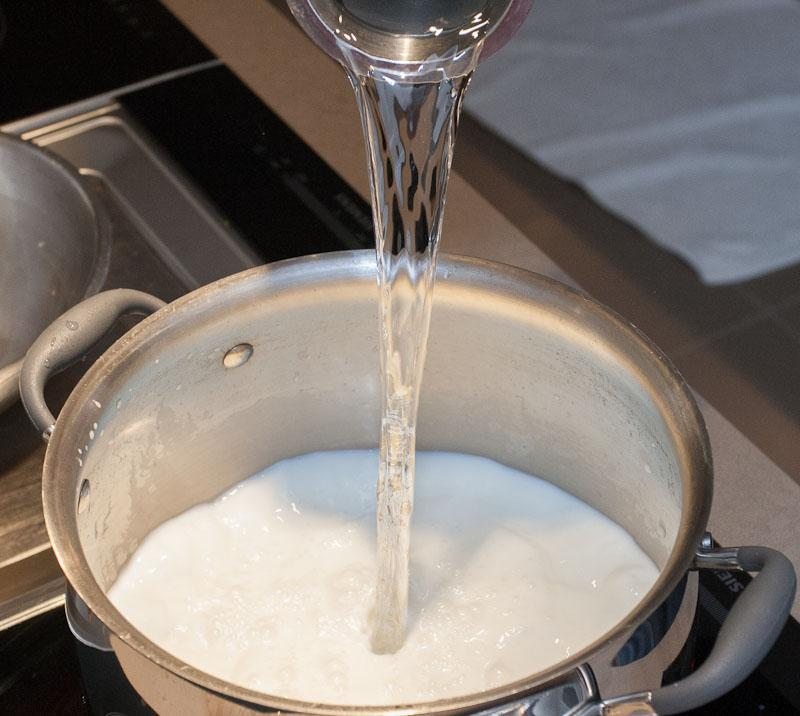# Arithmetic

#### Simple Interest & Compound Interest - 1

Profit and Loss : 2

Profit and Loss Continued:

Mark Up Price & Discount:Mark Up refers to the value an entity adds to the cost price of an article. Mark up is the price listed on the tag of an article. It is also known as List Price.

Discount refers to the rebate a shopkeeper offers to the customer.

For example: Let’s say the cost price of a watch is Rs 800 and the mark up price is Rs 1000. So, the shopkeeper has marked up the price of the watch by Rs 200. If let’s say he gives a discount of Rs 100 to the customer, so selling price of the watch or the price that the customer pays to the shopkeeper becomes Rs 900. So, eventually the profit earned by the shopkeeper becomes 1000-900 = Rs 100.

Now, some of the terms are defined as below:This can be solved with the help of successive change formula:

Profit% = Mark Up% - Discount% - Mark Up % *  Discount %100

Profit % = 25 – 10 – (25*10/100)

= 12.5%

Successive Discounts:

Sometimes, a series of discounts is offered and we call them successive discounts.

For example, if a discount of 30% +40% is offered, then it doesn’t imply a discount of 70%. In fact, first a discount of 30% is offered and then a discount of 40% is offered. Here, again the formula of successive discounts can be applied. Remember, we put a negative sign in front of the discount % value

So, effective discount becomes = -30 – 40 + (30*40)/100 = -58%

Negative sign here means that it is an overall discount of 58%.

So, in general if a successive discount of a% and b% is offered, then the overall discount becomes:  -a – b + (ab/100)

Now, let’s say that Colgate is giving an offer of 12.5% extra on its tooth paste. How much percent discount is the customer getting?

So, if initially a tooth paste weighs 8 grams, then the customer is now getting 9 grams for the cost of 8 grams. So, effectively he is getting a discount of 1 gram on 9 grams.

So, equivalent discount percent = (1/9) *100 = 11.11%

Example 1: A shopkeeper gives a discount of 10% on an article and thereby gains 20% in the transaction. What is the mark up%?

Solution: Here discount % = 10%, profit % = 20%, let the mark up % = a

So, 20 = a – 10 – (10a/100)

30 = 9a/10

or a = 300/9 = 100/3 = 33.33%

Example 2: A jeweler offers a discount of 15% to its customer and still makes a profit of 6.25%. Arvind bought a gold chain which was marked at Rs 5000. Find the cost price of the chain for the jeweler.

Solution: M.P. of the chain was Rs 5000,

Discount offered = 0.15* 5000 = Rs 750

SP = 5000 -750 = Rs 4250

Profit % = 6.25

CP = 4250/1.625 = Rs 4000

Example 3: The marked price of an article is 50% above the cost price. When marked price is increased by 20% and the selling price increased by 20%, the profit doubles. If the original marked price is Rs 300, then the original selling is-

Solution: As the marked price is 50% above the cost price and the original marked price is Rs 300, so the original CP becomes Rs 200

Let the original SP = Rs x

So, initial profit = x -200

New S.P. becomes 1.2x

New profit = 1.2x – 200

New profit = 2* Initial Profit

1.2x – 200 = 2(x-200)

1.2x – 200 = 2x – 400

200 = 0.8x

Or x = Rs 250

Case of faulty weights:Sometimes a shopkeeper uses faulty weights to increase his profit margin. For example if he uses 10% lesser weight, that means the actual weight is 10% lesser than 1000 grams i.e 900 grams. So, in a way he is charging for 1000 grams but is actually giving only 900 grams to the customer. Thus, this is a mark up for the shopkeeper. Mark up % = (100/900 )* 100 = 11.11% which is also equal to profit gained by the shopkeeper.

Now let’s say that a shopkeeper is using faulty weight which weighs 800 grams instead of 1000 grams. Further he increases the price of the commodity by 20%. How much percentage of profit is incurred by the shopkeeper?

So, by giving only 800 grams instead of 1000 grams, the shopkeeper has done a mark up of (200/800) *100 = 25%.

Further there is a mark up of 20%

So overall mark up % = Profit % = 25 + 20 +(25*20/100) = 50%

This problem can also be solved as:

Let the CP of 1 gram = 1 Re

So, the cost price of 800 grams = Rs 800

Also, he is charging Rs 1200 for 1000 grams. So, SP = Rs 1200

Thus, profit % = (400/800)*100 = 50%

Let’s do a twist in the above problem. Now, the shopkeeper is offering a discount of 10% and all the other things remain same. What is the new profit percentage?

As calculated from the earlier problem, the overall mark up done by the shopkeeper is 50%. Now, a discount of 10% is offered. Using successive change formula, the profit percentage becomes:

Profit % = 50 – 10 – (50*10/100) = 35%Sometimes, shopkeepers and retailers add impurities to the commodities to increase their profit percentage.

Let’s say a shopkeeper adds 20% impurities to sugar. How much percentage of mark up has the shopkeeper done?

So, if the quantity of pure substance is 5kg, the shopkeeper has added 1/5 i.e. 1 kg of impurity. So, out of 5kg pure sugar, 1 kg is the impurity. This is equivalent to a mark up of (1/5)*100 = 20%

In general if a shopkeeper adds a% impurity externally, then it is equivalent to a mark up of 20%

Let’s say that 20% of impurity is already present in the commodity. So, in every 5 kg of commodity, 1 kg is impurity and 4 kg of pure substance. Thus, it is equal to a mark up of (1/4)*100 = 25%.

Example 4: A dishonest dealer sells rice in which he weighs 20% lesser weight and also adds 25% impurity to the rice. What is the overall profit made by the dealer?

Solution: The dealer sells 20% lesser weight, this implies 25% mark up (from 1000 gram, actually the dealer is selling 800 gram, so this is a mark up of 25%)

Further, adding 20% impurities externally, is again a mark up of 20%.

So, overall mark up = 25 + 20 + (25*20/100)

= 50%

This, overall mark up is equivalent to the profit percentage earned by the dealer.

Example 5 :  Let a dishonest shopkeeper sells sugar at Rs 18/kg which he has bought at Rs 15/kg and he is giving 1000gm instead of 1200gm. Find his actual profit percentage.

Solution:  cost price of the sugar = Rs 15/kg

The selling price = Rs 18/kg.

Profit %= 18-1515*100= 20%

He is giving 1000gms instead of 1200gmsExample 6: What is the actual percentage gain when a dishonest shopkeeper, using a faulty balance makes a profit of 10% while buying as well as while selling his goods.

(a) 18.25
(b) 20
(c) 20.25
(d) 21

Solution: actual gain percentage= (10+10+10*10100)%

= 21%

Example 7: A dishonest milkman mixes water which costs nothing to milk costing Rs. 44 per litre to make profit of 25% on cost, by selling the resultant solution of milk and water for Rs. 50, the milkman wants to make profit, in what ratio should he mix water and milk.

Solution: Cost needed to net a 25% profit:

SP = 50

CP = 501.25=40

Actual cost: 44

Solution (x=liters of water needed to be added to the 1liter of milk):

(1+x) 40 = 44

x = 440=110

So, Milk: Water

1:1/10

So Milk: Water= 10:1

Example 8 : A dishonest dealer uses faulty weight and buys rice and does cheating of 10% while buying and 20 % while selling it to the customer. What is the profit percentage he makes in the entire transaction?

Solution: let us assume that the dealer brought 1000gms of rice for 1000rs and bought 10% rice more, which means he brought 1100gms for 1000 Rs.

CP of 1gm = 1011

He does cheating of 20%, this means he is selling 800gms of rice for 1000Rs

SP=1000Expansion and Contraction of Meter Scale:

It is a natural phenomenon that things expand in summers and contract in winters. But how does this affect a shopkeeper?

Let’s say we have a meter scale i.e. a scale with 100 cm. Now if this scale expands by let’s say 10%, so a customer would be getting 110 cm of cloth instead of 100 cm. So, here the shopkeeper is losing 10cm which is in a way a discount of (10/110)* 100 = 9.09% given to the customer.

If in the winters, the meter scale expands by 10%, then actually the shopkeeper is giving 90 cm of cloth and the customer is paying for 100 cm. This means that the shopkeeper is marking up the price by (100/900)*100 = 11.11%

Example 9: In the summers the meter scale expands by 20%. By how much percent must the shopkeeper mark up the price of the cloth, so that he gains 10% on the entire transaction?

Solution: If the meter scale expands by 20%, so the customer is getting 120 cm at the price of 100 cm.

So, equivalent discount = (20/120) * 100 = 16.66%

Let the mark up on the price by a%

Profit % = Mark Up% - Discount% - (MarkUp% * Discount %)/100

10 = a – 16.66 – (a*16.66)/100

Thus, a = 32%

So, the mark up on the price must be 32%